WAVEFORMS AND WAVE GENERATORS generator. Explain how the jump voltage is produced in a trapezoidal wave generator. ">Custom SearchWAVEFORMS AND WAVE GENERATORS LEARNING OBJECTIVES Upon completion of this chapter you will be able to: Explain the operation of astable, monostable, and bistable multivibrators. Explain the operation of a blocking oscillator. Explain the operation of a sawtooth generator. Explain the operation of a trapezoidal wave generator. Explain how the jump voltage is produced in a trapezoidal wave generator. WAVEFORMS This chapter will present methods of generating waveforms. Before you begin to study how waveforms are generated, you need to know the basic characteristics of waveforms. This section will discuss basic periodic waveforms. PERIODIC WAVEFORMS A waveform which undergoes a pattern of changes, returns to its original pattern, and repeats the same pattern of changes is called a PERIODIC waveform. Periodic waveforms are nonsinusoidal except for the sine wave. Periodic waveforms which will be discussed are the sine wave, square wave, rectangular wave, sawtooth wave, trapezoidal wave, and trigger pulses. Sine Wave Each completed pattern of a periodic waveform is called a CYCLE, as shown by the SINE WAVE in figure 3-1, view (A).Sine waves were presented in NEETS, Module 2, Alternating Current and Transformers, Chapter 1. Figure 3-1. - Periodic waveforms.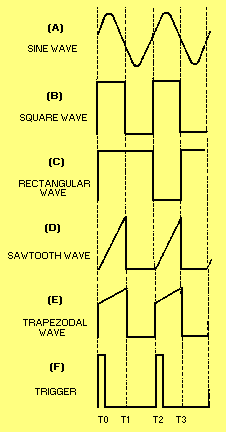Square Wave A SQUARE WAVE is shown in figure 3-1, view (B). As shown, it has two alternations of equal duration and a square presentation for each complete cycle. Figure 3-2 shows a breakdown of the square wave and is the figure you should view throughout the square wave discussion. The amplitude is measured vertically. The time for a complete cycle is measured between corresponding points on the wave (T0 to T2, or T1 to T3). Figure 3-2. - Square wave.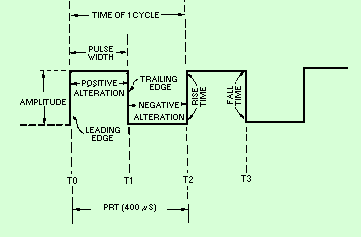One alternation is called a PULSE. The time for one complete cycle is called the PULSE-REPETITION TIME (prt). The number of times in 1 second that the cycle repeats itself is called the PULSE-REPETITION FREQUENCY (prf) or PULSE-REPETITION RATE (prr). If each alternation in figure 3-2 is 200 microseconds (ms), the prt will be 400 microseconds, and the prf will be 2,500 hertz. The following examples are provided to illustrate the mathematical relationship between prf and prt: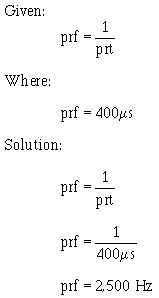You should readily see that prt is just the inverse of prf. Therefore: Given: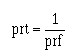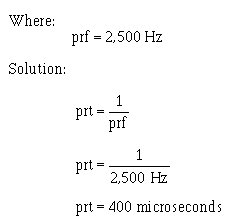The length of the pulse measured in time (T0 to T1) is referred to as the PULSE WIDTH (pw). The left side of the pulse is called the LEADING EDGE and the right side is called the TRAILING EDGE. Time is required for a voltage or current to change in amplitude. The interval of time needed for the voltage to go from 0 to 100 percent (or from 100 to 0 percent) of its maximum value is called the TRANSIENT INTERVAL. The two types of transient intervals are RISE TIME and FALL TIME. Rise time is more accurately defined as the time required for the voltage to build up from 10 percent to 90 percent of the maximum amplitude point. Fall time is the time required for the voltage to drop from 90 percent to 10 percent of the maximum amplitude point. In this text you will be presented with information in which waveforms appear to have instantaneous rise and fall times. This is done to simplify the presentation of the material. In reality these waveforms do have rise and fall times (transient intervals). Rectangular Wave A rectangular wave is similar to the square wave. The difference is that in the rectangular waveform, the two alternations of the waveform are of unequal time duration. Figure 3-1, view (C), shows that the negative alternation (pulse) is shorter (in time) than the positive alternation. The negative alternation could be represented as the longer of the two alternations. Either way, the appearance is that of a rectangle. Sawtooth Wave The SAWTOOTH waveform is shown in figure 3-1 , view (D). A sawtooth wave resembles the teeth of a saw blade. There is a rapid vertical rise of voltage from T0 to T1, which is linear (straight). At T1 this voltage abruptly falls (essentially no time used) to its previous static value. The voltage remains at this value until T2 when it again has a linear rise. You can see this action in an oscilloscope where there are two voltage input locations, vertical and horizontal. If you apply a linear voltage to the vertical input, the electron beam will be forced to move in a vertical direction on the crt. A linear voltage applied to the horizontal input will cause the electron beam to move horizontally across the crt. The application of two linear voltages, one to the vertical input and one to the horizontal input at the same time, will cause the beam to move in both a vertical and horizontal (diagonal) direction at the same time. This then is how a sawtooth wave is made to appear on an oscilloscope. You should refer to NEETS, Module 6, Electronic Emission, Tubes, and Power Supplies, Chapter 2, for a review of oscilloscopes. Trapezoidal Wave A TRAPEZOIDAL wave looks like a sawtooth wave on top of a square or rectangular wave, as shown in figure 3-1 , view (E). The leading edge of a trapezoidal wave is called the JUMP voltage. The next portion of the wave is the linear rise or SLOPE. The trailing edge is called the FALL or DECAY. A trapezoidal wave is used to furnish deflection current in the electromagnetic cathode ray tube and is found in television and radar display systems. Electromagnetic cathode ray tubes use coils for the deflection system, and a linear rise in current is required for an accurate horizontal display. The square or rectangular wave portion provides the jump voltage for a linear rise in current through the resistance of the coil. This will be explained further in a discussion of the trapezoidal sweep generator. Triggers A trigger is a very narrow pulse, as shown in figure 3-1 , view (F). Trigger pulses are normally used to turn other circuits on or off. WAVEFORM GENERATOR Nonsinusoidal oscillators generate complex waveforms such as those just discussed. Because the outputs of these oscillators are generally characterized by a sudden change, or relaxation, these oscillators are often called RELAXATION OSCILLATORS. The pulse repetition rate of these oscillators is usually governed by the charge and discharge timing of a capacitor in series with a resistor. However, some oscillators contain inductors that, along with circuit resistance, affect the output frequency. These RC and LC networks within oscillator circuits are used for frequency determination. Within this category of relaxation oscillators are MULTIVIBRATORS, BLOCKING OSCILLATORS, and SAWTOOTH- and TRAPEZOIDAL-WAVE GENERATORS. Many electronic circuits are not in an "on" condition all of the time. In computers, for example, waveforms must be turned on and off for specific lengths of time. The time intervals vary from tenths of microseconds to several thousand microseconds. Square and rectangular waveforms are normally used to turn such circuits on and off because the sharp leading and trailing edges make them ideal for timing purposes. MULTIVIBRATORS The type of circuit most often used to generate square or rectangular waves is the multivibrator. A multivibrator, as shown in figure 3-3, is basically two amplifier circuits arranged with regenerative feedback. One of the amplifiers is conducting while the other is cut off. Figure 3-3. - Astable Multivibrator.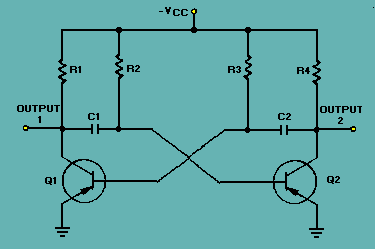When an input signal to one amplifier is large enough, the transistor can be driven into cutoff, and its collector voltage will be almost VCC. However, when the transistor is driven into saturation, its collector voltage will be about 0 volts. A circuit that is designed to go quickly from cutoff to saturation will produce a square or rectangular wave at its output. This principle is used in multivibrators. Multivibrators are classified according to the number of steady (stable) states of the circuit. A steady state exists when circuit operation is essentially constant; that is, one transistor remains in conduction and the other remains cut off until an external signal is applied. The three types of multivibrators are the ASTABLE, MONOSTABLE, and BISTABLE. The astable circuit has no stable state. With no external signal applied, the transistors alternately switch from cutoff to saturation at a frequency determined by the RC time constants of the coupling circuits. The monostable circuit has one stable state; one transistor conducts while the other is cut off. A signal must be applied to change this condition. After a period of time, determined by the internal RC components, the circuit will return to its original condition where it remains until the next signal arrives. The bistable multivibrator has two stable states. It remains in one of the stable states until a trigger is applied. It then FLIPS to the other stable condition and remains there until another trigger is applied. The multivibrator then changes back (FLOPS) to its first stable state. Q.1 What type circuit is used to produce square or rectangular waves?Q.2 What type of multivibrator does not have a stable state?Q.3 What type of multvibrator has one stable state?Q.4 What type of multivibrator has two stable states?Integrated Publishing, Inc. - A (SDVOSB) Service Disabled Veteran Owned Small Business